### IMO Shortlist 1982 problem 17

Kvaliteta:
Avg: 0.0
Težina:
Avg: 0.0
The right triangles$ABC$ and$AB_1C_1$ are similar and have opposite orientation. The right angles are at$C$ and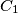$C_1$, and we also have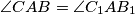$\angle CAB = \angle C_1AB_1$. Let$M$ be the point of intersection of the lines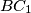$BC_1$ and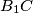$B_1C$. Prove that if the lines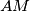$AM$ and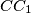$CC_1$ exist, they are perpendicular.
Source: Međunarodna matematička olimpijada, shortlist 1982